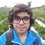# Pre-RMO 2014/5

If real numbers $a, b, c, d, e$ satisfy

$a + 1 = b + 2 = c + 3 = d + 4 = e + 5 = a + b + c + d + e + 3,$

what is the value of $a^2 + b^2 + c^2 + d^2 + e^2$?

This note is part of the set Pre-RMO 2014Note by Pranshu Gaba
6 years, 8 months ago

This discussion board is a place to discuss our Daily Challenges and the math and science related to those challenges. Explanations are more than just a solution — they should explain the steps and thinking strategies that you used to obtain the solution. Comments should further the discussion of math and science.

When posting on Brilliant:

• Use the emojis to react to an explanation, whether you're congratulating a job well done , or just really confused .
• Ask specific questions about the challenge or the steps in somebody's explanation. Well-posed questions can add a lot to the discussion, but posting "I don't understand!" doesn't help anyone.
• Try to contribute something new to the discussion, whether it is an extension, generalization or other idea related to the challenge.
• Stay on topic — we're all here to learn more about math and science, not to hear about your favorite get-rich-quick scheme or current world events.

MarkdownAppears as
*italics* or _italics_ italics
**bold** or __bold__ bold
- bulleted- list
• bulleted
• list
1. numbered2. list
1. numbered
2. list
Note: you must add a full line of space before and after lists for them to show up correctly
paragraph 1paragraph 2

paragraph 1

paragraph 2

[example link](https://brilliant.org)example link
> This is a quote
This is a quote
    # I indented these lines
# 4 spaces, and now they show
# up as a code block.

print "hello world"
# I indented these lines
# 4 spaces, and now they show
# up as a code block.

print "hello world"
MathAppears as
Remember to wrap math in $$ ... $$ or $ ... $ to ensure proper formatting.
2 \times 3 $2 \times 3$
2^{34} $2^{34}$
a_{i-1} $a_{i-1}$
\frac{2}{3} $\frac{2}{3}$
\sqrt{2} $\sqrt{2}$
\sum_{i=1}^3 $\sum_{i=1}^3$
\sin \theta $\sin \theta$
\boxed{123} $\boxed{123}$

## Comments

Sort by:

Top Newest

Solving the equations a=2,b=1,c=o,d=-1,e=-2.Thus the answer is 10.

- 6 years, 8 months ago

Log in to reply

But their square is 0

- 2 years, 11 months ago

Log in to reply

let this all be equal to k a=k-1 ,b=k-2 ,c=k-3, d=k-4 ,e=k-5 now substituting these values in last equation we get a,b,c,d,e

- 6 years, 8 months ago

Log in to reply

I did this sum by solving each part individually i.e I obtained every part in terms of $d$ and then I solved the equation to get the answer as 10.

- 6 years, 6 months ago

Log in to reply

10

- 6 years, 1 month ago

Log in to reply

$\color{#D61F06}{\boxed{10}}$

- 5 years, 10 months ago

Log in to reply

10

- 5 years, 10 months ago

Log in to reply

10 😊

- 5 years, 10 months ago

Log in to reply

let this all be equal to k a=k-1 ,b=k-2 ,c=k-3, d=k-4 ,e=k-5 now substituting these values in last equation we get a,b,c,d,e

- 6 years, 8 months ago

Log in to reply

10

- 6 years, 8 months ago

Log in to reply

10

- 6 years, 8 months ago

Log in to reply

the answer is 10..

- 6 years, 8 months ago

Log in to reply

Equate everything to k and substitute accordingly.The answer is 10.

- 6 years, 8 months ago

Log in to reply

10

- 6 years, 8 months ago

Log in to reply

10

- 6 years, 8 months ago

Log in to reply

×

Problem Loading...

Note Loading...

Set Loading...